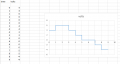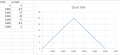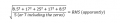# question from book, rms

#### ninjaman

Joined May 18, 2013
309
hello,

i am reading a book called "electronic principles and applications" by john pratley. there is a question in the exercise part that i am stuck on.
6. a steeped a.c wave has teh following values over equal intervals of time
value 4 6 6 4 2 0 0 -2 -4
time 0-1 1-2 2-3 3-4 4-5 5-6 6-7 7-8 8-9

I thought that the rms of a sine wave, not that this is a sine wave, would be 0.707 x max value, 0.707 x 6 which is not the answer. i looked at the answer which is 3.74 and * 1.414 to get 5.28.i did this in excel to help me find the answer.
the exercise questions cover information from chapters: peak to peak values, average or mean value of an alternating waveform, rms of an alternating waveform.
some of the questions in the exercises are a little confusing as none of the information has been covered yet. i also googled this question and found that it was taken from a different book by a different writer, or the other way round (plagarism)

#### DGElder

Joined Apr 3, 2016
351
rms stands for root mean square. You find the mean (average) of the squared values. Then you find the square root of that mean. For a stepped function of equal time increments, e.g. 4, 3 ,0 -2. => rms = Square Root [( 16+9+0+4)/4] = 2.7

Multiplying the rms value by 1.414 is meaningless since it is not a sinewave.

•ninjaman

#### DGElder

Joined Apr 3, 2016
351
That's wrong, you need to use the whole signal, or one cycle of the signal if it is periodic, to determine the rms value. If the negative values are identical, i.e. a reflection of the positive values, then you could get the same answer by just performing the rms calculation on the positive values - or just on the negative values.

Last edited:

#### Veracohr

Joined Jan 3, 2011
688
from what i have read in the book it says to take the values from half of the wave, the positive half.
That's only applicable if the signal is perfectly symmetrical about zero. If that is the case, then the sum of the positive values squared will be equal to the sum of the negative values squared. But if your signal goes from -2V to +5V then the two polarities aren't equal and the RMS value changes because the sum of the squared values changes.

#### WBahn

Joined Mar 31, 2012
24,700
from what i have read in the book it says to take the values from half of the wave, the positive half. so i took 4, 6, 6, 4, 2.
i put these in a calculator
View attachment 107764
if i included the two zeros i would have 108 over 7 (all square rooted) = 3.93
the book says 3.74
Your main problem is that you are looking for some formula to just blindly use without being willing to understand where those formulas come from and, hence, what their limitations are.

The concept of the RMS value of a waveform is very simple.

Apply that voltage waveform to a resistor (you can use a generic, symbolic value such as R or you can pick an arbitrary value such as 1 Ω). Determine what the average power is that is being dissipated in that resistor. Then simply determine what DC voltage value would dissipate that same power in that same resistor. That value is, by definition, the effective voltage of the waveform, which we call the RMS value because of the mathematical steps it turns out need to be followed to calculated it.

Since power is the rate of energy transfer, the average power over some time interval is going to be the total energy transferred divided by the time it too to do so.

During a period of time, T, in which the voltage, v(t), is constant, the energy dissipated in a resistor, R, by that voltage is

$$E \; = \; \frac{v^2(t)}{R}T$$

So go through all nine time intervals and calculate the energy in each time interval and add them all up. Now divide by the total length of time (9T). That will give you the average power. Now simply use the relationship P = V²/R to find the value of V that will dissipate that same power in that same R.

#### MrAl

Joined Jun 17, 2014
6,500
hello,

i am reading a book called "electronic principles and applications" by john pratley. there is a question in the exercise part that i am stuck on.
6. a steeped a.c wave has teh following values over equal intervals of time
value 4 6 6 4 2 0 0 -2 -4
time 0-1 1-2 2-3 3-4 4-5 5-6 6-7 7-8 8-9

I thought that the rms of a sine wave, not that this is a sine wave, would be 0.707 x max value, 0.707 x 6 which is not the answer. i looked at the answer which is 3.74 and * 1.414 to get 5.28.
View attachment 107761
i did this in excel to help me find the answer.
the exercise questions cover information from chapters: peak to peak values, average or mean value of an alternating waveform, rms of an alternating waveform.
some of the questions in the exercises are a little confusing as none of the information has been covered yet. i also googled this question and found that it was taken from a different book by a different writer, or the other way round (plagarism)

Hello there,

The answer you have so far, 3.74, is very close to the right result but there is a better result when rounded to three figures like that. The more accurate result is 3.7x and i'll leave off that last digit for now.

There is a formula for calculating the RMS value piece wise which everyone seems to be trying to accomplish here. The idea is to solve for one piece of the wave and then use that in such a way as to get the result for the entire wave.
So you can check your answers a little better, the piecewise formula is:
Vn=|E|*sqrt(T/Tp)

where
Vn is the partial voltages to be found first,
E is the peak voltage for the rectangular section being done,
T is the time of that section,
Tp is the total time of the entire wave.

The total RMS value is then:
Vrms=sqrt(sum of all Vn^2)=sqrt(V1^2+V2^2+V3^2+...Vn^2)

Since all of the values are squared, it is convenient to use the form:
Vrms=sqrt(sum of all Vm)
where
Vm=E^2*T/Tp

So for example Vm for one section that is 6v would be:
V1=36*1/9

and since we have made T=1 there we have to include that twice V1+V1 or else use:
V1=36*2/9

Once you calculate all the Vm this way you can do:
Vrms=sqrt(sum of all Vm)

and that gives you the total RMS voltage.

Note again however that this applies only for rectangular pulses, although the summation idea may work for any kind of partial waveform (we could check into this).

You may also want to note that the definition of RMS is:
Vrms=sqrt[(1/Tp)*integral(E^2) {from 0 to T} dt]
where
Tp is the total period,
T is the time of the pulse,
E is the peak voltage.

The reason why the formula comes out so simple for the rectangular pulses is because the integration of a rectangular pulse comes out simple.

You should use whatever method you started with and then compare to these results.
The more accurate result is between 3.70 and 3.80 but i'll wait till you try this again to post the exact result.

#### ninjaman

Joined May 18, 2013
309
i have this as a question in the book i am reading. there is no mention of calculus or time and i am only trying to learn what the book is telling me. but for some reason i cannot get the correct answer.the values are provided and i have to create a graph which i have done. i imagine it is symmetrical about zero.

That's only applicable if the signal is perfectly symmetrical about zero. If that is the case, then the sum of the positive values squared will be equal to the sum of the negative values squared.
i have put this into a calculator, square root first, then putting the squared values added over the number of values and i get 13.87.
i have tried to follow along with the examples, inserting these new values and leaving out the zeros but no luck.
i am currently reading a "teach yourself algebra" book by p. abbot, and a book about trigonometry by chris mcmullen. also, i have a book about calculus called "quick calculus" by daniel kleppner. neither of these books mention mid ordinate or current/voltage in a sine wave. the trig book talks about sine waves but not this. the only book that explains this mid ordinate stuff is the book i have at the moment. there is no mention of calculus in this book. i am just having a problem working my way through it.
any help is appreciated.
thanks

#### DGElder

Joined Apr 3, 2016
351
I explained how to do it in post #2. That is not what you did. What is the first thing I said you should do?

This is not symmetric about zero current, it is however symmetric about 0.003 time. But you don't need to utilize that to solve the problem.

#### WBahn

Joined Mar 31, 2012
24,700
i have this as a question in the book i am reading. there is no mention of calculus or time and i am only trying to learn what the book is telling me. but for some reason i cannot get the correct answer.
View attachment 108033
the values are provided and i have to create a graph which i have done. i imagine it is symmetrical about zero.

i have put this into a calculator, square root first, then putting the squared values added over the number of values and i get 13.87.
i have tried to follow along with the examples, inserting these new values and leaving out the zeros but no luck.
i am currently reading a "teach yourself algebra" book by p. abbot, and a book about trigonometry by chris mcmullen. also, i have a book about calculus called "quick calculus" by daniel kleppner. neither of these books mention mid ordinate or current/voltage in a sine wave. the trig book talks about sine waves but not this. the only book that explains this mid ordinate stuff is the book i have at the moment. there is no mention of calculus in this book. i am just having a problem working my way through it.
any help is appreciated.
thanks
This is a different problem, so I first want to make sure what you are trying to find. Are you supposed to find the RMS current for this waveform?

You're sure that the book that this was taken from has not mentioned integration at all with regards to RMS values up to this point?

Given the data values you have, you can only find the RMS value over the period of time that the waveform is defined, namely from t=0 to t=0.006 s (I'm assuming that time is in seconds and the current is in amperes).

You need to calculated RMS as the ROOT of the MEAN of the SQUARE. So first you need to SQUARE the waveform, then you need to calculate the MEAN (the average) of the squared waveform, then you need to take the square ROOT of that average. While you can do this numerically, you need to have a lot more points than what you have in order to get a good estimate. The best way is to first determine a suitable functional relationship between the current and time. In this case this is very easy to do for the rising part of the waveform. Since the waveform is symmetric about t = 3 ms, we know that the RMS value of the first half of the waveform will be the same as the second which will, in turn, be the same as the overall waveform. So we only need to find the RMS value from t = 0 to t = 3 ms.

The equation that describes the rising part of the waveform is

i(t) = a·t

where

a = (25/3) A/ms

So square that waveform, integrate it from t = 0 to t = 3 ms, divide by 3 ms to get the mean, and then take the square root of that.

#### ninjaman

Joined May 18, 2013
309
no, there is no integration in the book and i do not know how to do that yet. the formula in the book is a square root or radical (i think it is called) with a fraction in it. on the top of the fraction are the values, each squared and added to the next. on the bottom of the fraction is the number of values.this is the formula in the book. no real explanation of mid ordinates, just a few examples that dont make a lot of sense.
I explained how to do it in post #2. That is not what you did. What is the first thing I said you should do?
so i get 1347.5, this is the values of voltage, over 5, the number of values i get 269.5. i take this as the mean of the squared values. i then find the root by putting this under the square root to get 16.42

#### WBahn

Joined Mar 31, 2012
24,700
If that is how that book is telling you to compute RMS, then you need to get a different book.

#### The Electrician

Joined Oct 9, 2007
2,737
no, there is no integration in the book and i do not know how to do that yet. the formula in the book is a square root or radical (i think it is called) with a fraction in it. on the top of the fraction are the values, each squared and added to the next. on the bottom of the fraction is the number of values.
View attachment 108051
this is the formula in the book. no real explanation of mid ordinates, just a few examples that dont make a lot of sense.

so i get 1347.5, this is the values of voltage, over 5, the number of values i get 269.5. i take this as the mean of the squared values. i then find the root by putting this under the square root to get 16.42
You are doing an approximate numerical integration and the result will only be approximate. The accuracy of the result will increase as you subdivide the interval into more pieces.

Using the abscissae of your approximating rectangle as .001, .002 and .003 successively leaves out the area around zero.

It will be better to use as midpoints .0005, .0015, and .0025. Then for a better result, use twice as many, namely .00025, .00075, .00125, etc.

#### WBahn

Joined Mar 31, 2012
24,700

#### DGElder

Joined Apr 3, 2016
351
no, there is no integration in the book and i do not know how to do that yet. the formula in the book is a square root or radical (i think it is called) with a fraction in it. on the top of the fraction are the values, each squared and added to the next. on the bottom of the fraction is the number of values.this is the formula in the book. no real explanation of mid ordinates, just a few examples that dont make a lot of sense.

I explained how to do it in post #2. That is not what you did. What is the first thing I said you should do?

so i get 1347.5, this is the values of voltage, over 5, the number of values i get 269.5. i take this as the mean of the squared values. i then find the root by putting this under the square root to get 16.42

As I understand it, you were given those values 0, 8.5, 17, etc. You created the graph (labeled "Chart Title"), which misrepresents the problem as it should show a stepwise function of equal time increments. Then the formula from the book is correct when including the zeros and dividing by 7 (not 5) inside the radical - which gives rms = 13.87 A, mA or whatever are the units for the current.

Last edited: